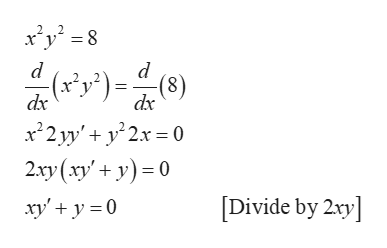# Use implicit differentiation to find y’’ of the equation x^2y^2=8.

Question
1 views

Use implicit differentiation to find y’’ of the equation x^2y^2=8.

check_circle

Step 1

Differentiate as...help_outlineImage Transcriptionclosexy=8 2 2 d d (x x22yy'2x 0 2ry (xy'y0 'y')= (8) dx dx [Divide by 2xy xy'y0 fullscreen

### Want to see the full answer?

See Solution

#### Want to see this answer and more?

Solutions are written by subject experts who are available 24/7. Questions are typically answered within 1 hour.*

See Solution
*Response times may vary by subject and question.
Tagged in

### Derivative